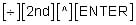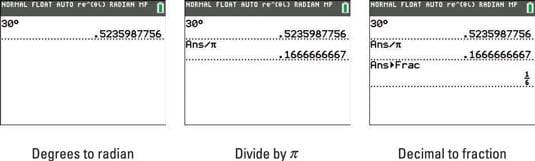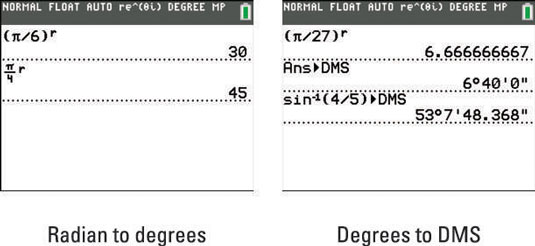##### TI-84 Plus CE Graphing Calculator For DummiesThe functions housed in the Angle menu on your TI-84 Plus calculator enable you to convert between degrees and radians or convert between rectangular and polar coordinates. To convert degrees to radians, follow these steps:
1. Put the calculator in Radian mode.

Press [MODE], use the arrow keys to highlight RADIAN, and then press [ENTER].

2. If necessary, press [2nd][MODE] to access the Home screen.

3. Enter the number of degrees.

4. Press [2nd][APPS] to paste in the degree function.

5. Press [ENTER] to convert the degree measure to radians.

This is illustrated in the first screen.

If you’re a purist who likes to see radian measures expressed as a fractional multiple of pi whenever possible, continuing with the following steps accomplishes this goal if it’s mathematically possible.

6. To divide the radian measure by pi, pressThis is illustrated in the second screen.

7. Press [MATH][ENTER][ENTER] to convert the result to a fraction, if possible.

This is illustrated in the third screen. If the calculator can’t convert the decimal obtained in Step 6 to a fraction, it says so by returning the decimal in Step 7.## To convert radians to degrees:

1. Put the calculator in Degree mode.

Press [MODE], use the arrow keys to highlight DEGREE, and then press [ENTER].

2. If necessary, press [2nd][MODE] to access the Home screen.

If the radian measure is entered as an arithmetic expression, surround that expression with parentheses.

4. Press [2nd][APPS] to paste in the r function.

5. Press [ENTER] to convert the radian measure to degrees.

This is illustrated in the first screen.The TI-84 Plus is a complicated tool and it's easy to make mistakes on it. For more help, check out 10 Common Errors When Using the TI-84 Plus Calculator.